# Jacobi polynomials

Jump to: navigation, search

Orthogonal polynomials on the interval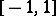with the weight function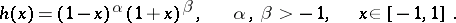The standardized Jacobi polynomials are defined by the Rodrigues formula: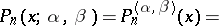and the orthonormal Jacobi polynomials have the form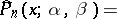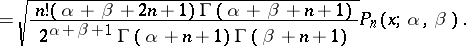The polynomialsatisfies the differential equationWhen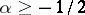and, the orthonormal Jacobi polynomials satisfy the following weighted estimate: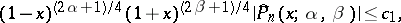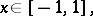where the constant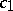does not depend onand. Atthe sequencegrows at a rate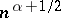and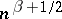, respectively.

Fourier series in Jacobi polynomials (cf. Fourier series in orthogonal polynomials) inside the intervalare similar to trigonometric Fourier series. But in neighbourhoods of the end points of this interval, the orthogonality properties of Fourier–Jacobi series are different, because at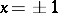the orthonormal Jacobi polynomials grow unboundedly. The Fourier–Jacobi series of a functionis uniformly convergent onifistimes continuously differentiable on this segment and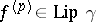with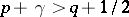, where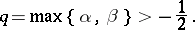Under these conditions the following inequality holds: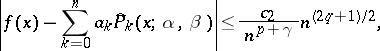where the constant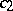does not depend onand. On the other hand, whenand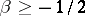, the remainder in the Fourier–Jacobi series forsatisfies the following weighted estimate: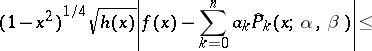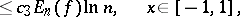where, the constantdoes not depend onand, and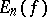is the best uniform approximation error (cf. Best approximation) of the continuous functiononby polynomials of degree not exceeding.

The Jacobi polynomials were introduced by C.G.J. Jacobi  in connection with the solution of the hypergeometric equation. Special cases of the Jacobi polynomials are: the Legendre polynomials (when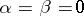); the Chebyshev polynomials of the first kind (when); the Chebyshev polynomials of the second kind (when); and the ultraspherical polynomials (when).

See also Classical orthogonal polynomials.